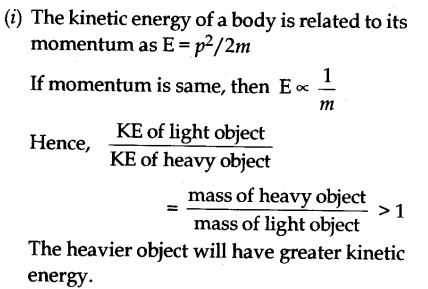# (i) A light and a heavy object have the same momentum. What is the ratio of their kinetic energies. Which one has a larger kinetic energy?

(i) A light and a heavy object have the same momentum. What is the ratio of their kinetic energies. Which one has a larger kinetic energy ?
(ii) A ball is dropped from a height of 10 m. If the energy of the ball reduces by 40% after striking the ground, how much high can the ball bounce back ? (g = 10m s^-2)(ii) Given h1 = 10 m,
E1 = E,
E2 = 0.6 (reduced by 40 %),
h2 = ?
Therefore,
mgh2 = 0.6 mgh1 or
h2 = h1 x 0.6 = 6 m